### How to calculate Slope in Excel

In this Article we will learn to calculate the slope in Excel.

## Finding slope using Excel function

Les us try to calculate the slope using simple formula’s by using the coordinates of 2 points. First write the coordinates of 2 points: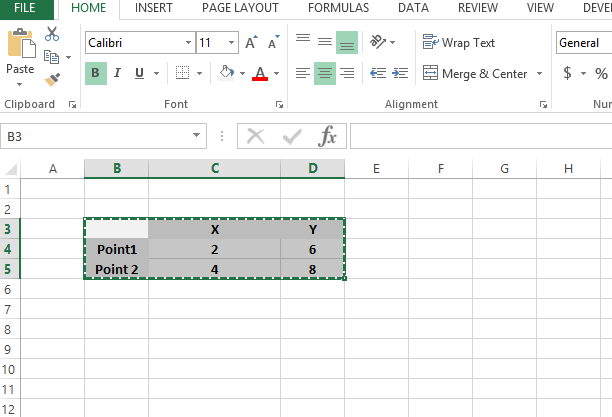Calculate difference between x coordinates: C5-C4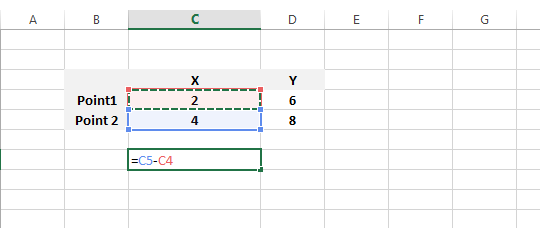The result is 2 in this example.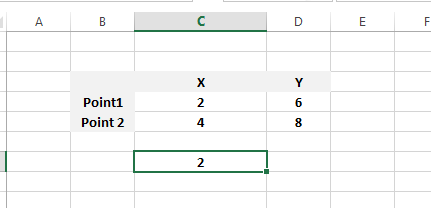Now let us calculate difference between y coordinates: D5-D4The result is 2 again.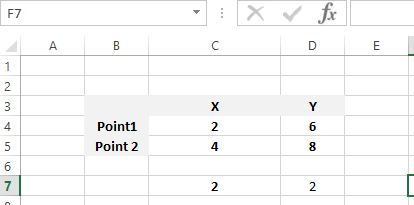Slope will be difference of Y coordinates divided by difference of X coordinates:The Slope here is 1.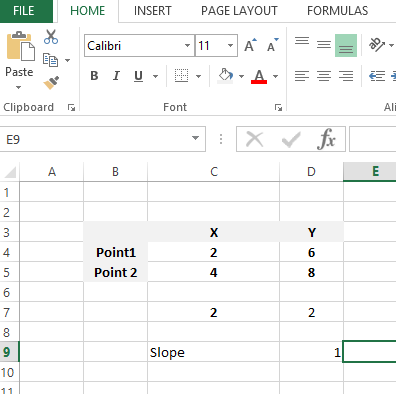This was very simple approach, now we will do the same by using the slope function inbuilt in Excel:

SLOPE(known_y’s, known_x’s)

The SLOPE function syntax has the following arguments:

• Known y’s Required. An array or cell range of numeric dependent data points.
• Known x’s Required. The set of independent data points.See in this example as we are typing the formula it is being guided by Excel with the comments below. The slope formula written as SLOPE(KNOWN Y’S,KNOWN X’S)

So the formula will be:

=SLOPE(D4:D5,C4:C5)

The final contents in the sheet with results is like:## Finding slope from the chart

There is no need to use slope formula to find the slope. Sometimes it might be possible to find it from the graph.

Lets assume we have such data.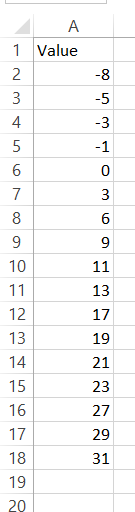There is a measurement of some value. And you need to find the slope for the value. How to do that?

First plot the line chart for that. Go to Insert – Line Chart.You can see the simple chart.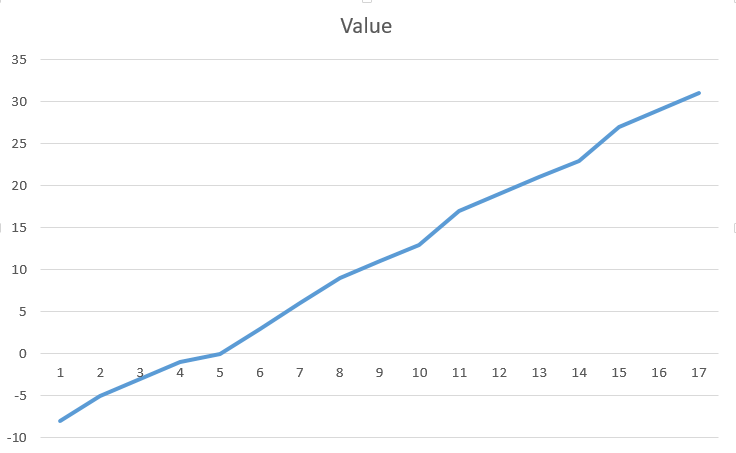Right click the line and choose Add Trendline.Menu pops in the right side of your screen. Choose “Display Equation on the Chart”.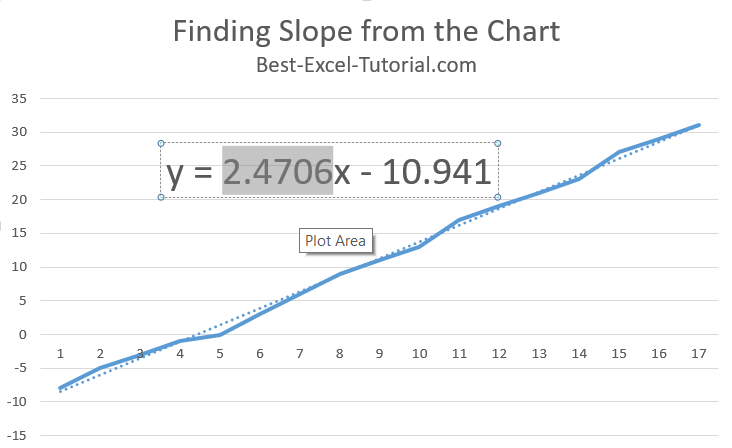Can’t you see that? If you forgot I will remind.

The equation of the trendline comes from the y = f(x) = ax + b

In this example y = 2.4706x – 10.941

a = 2.4706 and b = -10.941

a = slope = 2.4706

Note: b is the point where the line cross the horizontal line for x=0 point.
To continue pls check how to insert Slope Graph tutorial.

## Template

```Further reading: